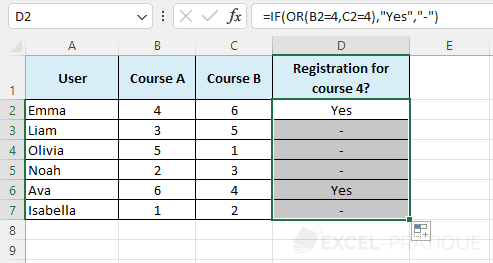# Excel Function: OR

The Excel function OR checks if at least one of the tests is TRUE, it returns FALSE only if all tests are FALSE.

This function is generally used in combination with other functions (as in the following example with the function IF).

Usage:

`=OR(test_1, test_2, etc)`

## Example of use

The goal here is to check if the user is registered for course 4 by testing IF the value of the cell in column B OR column C is equal to 4: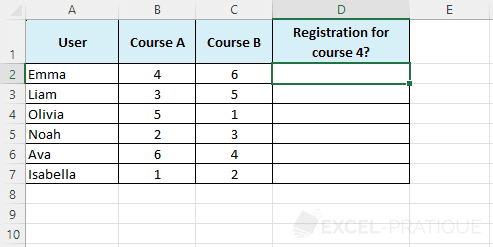Start by selecting the IF function and enter the Value_if_true and Value_if_false: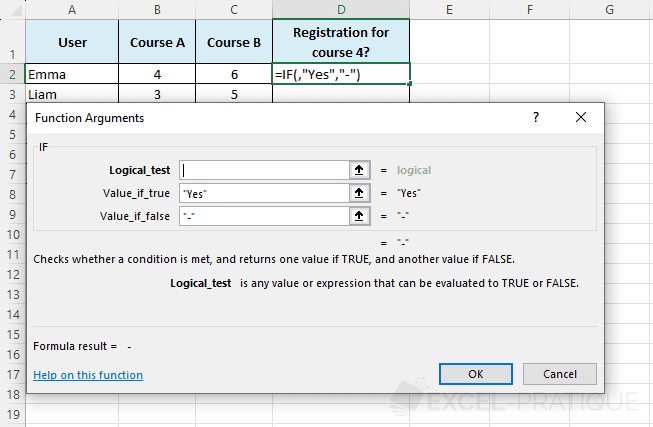Now click in Logical_test and select the OR function from the drop-down list: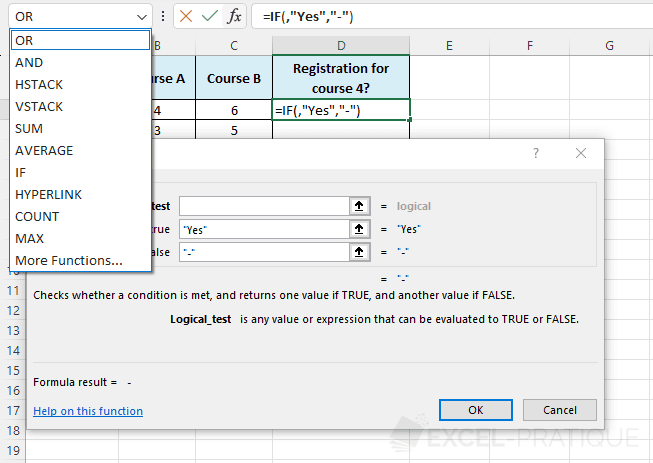Finally, enter the tests to be performed: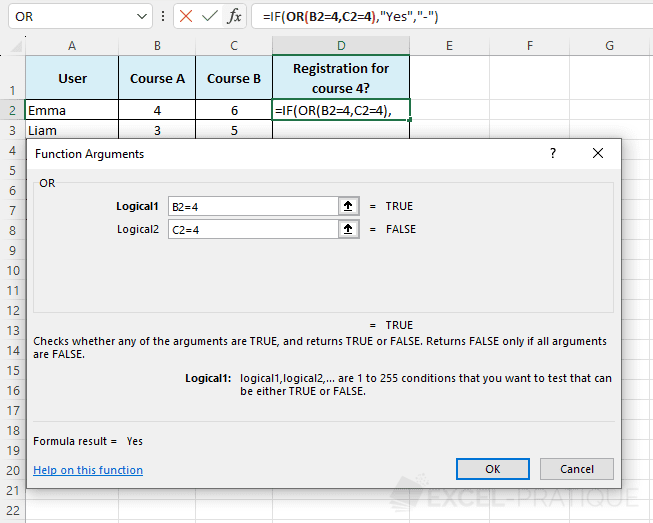The formula therefore returns "Yes" because at least one of the tests is TRUE:

``=IF(OR(B2=4,C2=4),"Yes","-")``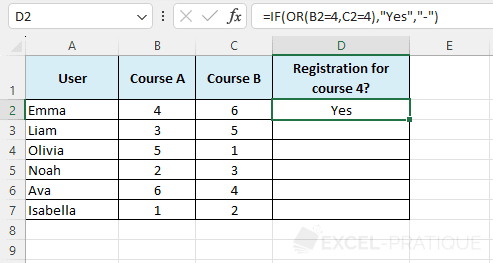Then stretch the formula to apply it to the other cells: